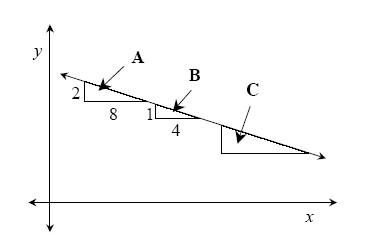### Home > GC > Chapter 3 > Lesson 3.2.6 > Problem3-98

3-98.

Examine the graph of the line at right.1. Find the slope of this line using slope triangle $A$.

How do you find the slope of a line?

1. Find the slope using slope triangle $B$.

See part (a).

1. Without calculating, what does the slope ratio for slope triangle $C$ have to be?

If the triangle is on the same line, what does that tell you about the slope?